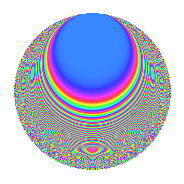# Properties

 Label 8015.2.a.kLevel 8015 Weight 2 Character orbit 8015.a Self dual Yes Analytic conductor 64.000 Analytic rank 1 Dimension 49 CM No

# Related objects

## Newspace parameters

 Level: $$N$$ = $$8015 = 5 \cdot 7 \cdot 229$$ Weight: $$k$$ = $$2$$ Character orbit: $$[\chi]$$ = 8015.a (trivial)

## Newform invariants

 Self dual: Yes Analytic conductor: $$64.0000972201$$ Analytic rank: $$1$$ Dimension: $$49$$ Fricke sign: $$1$$ Sato-Tate group: $\mathrm{SU}(2)$

## $q$-expansion

The dimension is sufficiently large that we do not compute an algebraic $$q$$-expansion, but we have computed the trace expansion.

 $$\operatorname{Tr}(f)(q) =$$ $$49q - 3q^{2} - 10q^{3} + 49q^{4} - 49q^{5} + 10q^{6} - 49q^{7} - 6q^{8} + 39q^{9} + O(q^{10})$$ $$\operatorname{Tr}(f)(q) =$$ $$49q - 3q^{2} - 10q^{3} + 49q^{4} - 49q^{5} + 10q^{6} - 49q^{7} - 6q^{8} + 39q^{9} + 3q^{10} + 16q^{11} - 26q^{12} - 31q^{13} + 3q^{14} + 10q^{15} + 49q^{16} - 18q^{17} + 4q^{18} - 16q^{19} - 49q^{20} + 10q^{21} + 10q^{22} + 10q^{23} + 2q^{24} + 49q^{25} - 22q^{26} - 58q^{27} - 49q^{28} + 31q^{29} - 10q^{30} - 35q^{31} - 5q^{32} - 82q^{33} - 41q^{34} + 49q^{35} + 49q^{36} - 24q^{37} - 20q^{38} + 41q^{39} + 6q^{40} + 30q^{41} - 10q^{42} - 19q^{43} + 27q^{44} - 39q^{45} + 15q^{46} - 39q^{47} - 51q^{48} + 49q^{49} - 3q^{50} + 46q^{51} - 94q^{52} - 17q^{53} + 9q^{54} - 16q^{55} + 6q^{56} - 23q^{57} - 46q^{58} + 11q^{59} + 26q^{60} - 9q^{61} - 49q^{62} - 39q^{63} + 10q^{64} + 31q^{65} - 10q^{66} - 2q^{67} - 73q^{68} - 47q^{69} - 3q^{70} + 26q^{71} - 39q^{72} - 100q^{73} + 8q^{74} - 10q^{75} - 71q^{76} - 16q^{77} - 51q^{78} + 50q^{79} - 49q^{80} + 61q^{81} - 36q^{82} - 67q^{83} + 26q^{84} + 18q^{85} + 33q^{86} - 45q^{87} - q^{88} - 19q^{89} - 4q^{90} + 31q^{91} + 7q^{92} + 9q^{93} - 33q^{94} + 16q^{95} - 8q^{96} - 85q^{97} - 3q^{98} + 27q^{99} + O(q^{100})$$

## Embeddings

For each embedding $$\iota_m$$ of the coefficient field, the values $$\iota_m(a_n)$$ are shown below.

For more information on an embedded modular form you can click on its label.

Label $$a_{2}$$ $$a_{3}$$ $$a_{4}$$ $$a_{5}$$ $$a_{6}$$ $$a_{7}$$ $$a_{8}$$ $$a_{9}$$ $$a_{10}$$
1.1 −2.67776 −3.22300 5.17039 −1.00000 8.63041 −1.00000 −8.48955 7.38771 2.67776
1.2 −2.66490 2.35676 5.10167 −1.00000 −6.28053 −1.00000 −8.26563 2.55434 2.66490
1.3 −2.62540 −0.161903 4.89272 −1.00000 0.425059 −1.00000 −7.59456 −2.97379 2.62540
1.4 −2.56687 −1.38440 4.58882 −1.00000 3.55358 −1.00000 −6.64517 −1.08344 2.56687
1.5 −2.52338 −1.42453 4.36744 −1.00000 3.59462 −1.00000 −5.97394 −0.970719 2.52338
1.6 −2.33828 2.05266 3.46754 −1.00000 −4.79969 −1.00000 −3.43150 1.21342 2.33828
1.7 −2.29229 −2.84815 3.25461 −1.00000 6.52878 −1.00000 −2.87593 5.11193 2.29229
1.8 −2.08886 −0.324198 2.36335 −1.00000 0.677204 −1.00000 −0.758978 −2.89490 2.08886
1.9 −2.05694 0.523578 2.23099 −1.00000 −1.07697 −1.00000 −0.475131 −2.72587 2.05694
1.10 −1.92336 −1.33966 1.69931 −1.00000 2.57665 −1.00000 0.578330 −1.20531 1.92336
1.11 −1.91964 0.294978 1.68500 −1.00000 −0.566250 −1.00000 0.604679 −2.91299 1.91964
1.12 −1.80610 2.35882 1.26200 −1.00000 −4.26027 −1.00000 1.33289 2.56402 1.80610
1.13 −1.73153 −2.59444 0.998212 −1.00000 4.49236 −1.00000 1.73463 3.73110 1.73153
1.14 −1.56783 2.04149 0.458083 −1.00000 −3.20071 −1.00000 2.41746 1.16770 1.56783
1.15 −1.36146 −0.153831 −0.146418 −1.00000 0.209435 −1.00000 2.92227 −2.97634 1.36146
1.16 −1.23019 2.26268 −0.486637 −1.00000 −2.78352 −1.00000 3.05903 2.11970 1.23019
1.17 −1.12638 −2.96921 −0.731259 −1.00000 3.34447 −1.00000 3.07645 5.81619 1.12638
1.18 −0.978041 −3.27758 −1.04343 −1.00000 3.20561 −1.00000 2.97661 7.74254 0.978041
1.19 −0.788296 −1.23031 −1.37859 −1.00000 0.969852 −1.00000 2.66333 −1.48633 0.788296
1.20 −0.736107 −1.41103 −1.45815 −1.00000 1.03867 −1.00000 2.54557 −1.00899 0.736107
See all 49 embeddings
 $$n$$: e.g. 2-40 or 990-1000 Embeddings: e.g. 1-3 or 1.49 Significant digits: Format: Complex embeddings Normalized embeddings Satake parameters Satake angles

## Inner twists

This newform does not have CM; other inner twists have not been computed.

## Atkin-Lehner signs

$$p$$ Sign
$$5$$ $$1$$
$$7$$ $$1$$
$$229$$ $$1$$

## Hecke kernels

This newform can be constructed as the intersection of the kernels of the following linear operators acting on $$S_{2}^{\mathrm{new}}(\Gamma_0(8015))$$:

 $$T_{2}^{49} + \cdots$$ $$T_{3}^{49} + \cdots$$